# Z1 cross procedure (placing probe at center of shim stack)

Z1 cross procedure aims at placing probe coils at the center of the magnet. This goal is achieved indirectly by placing probe at the center of shim stack, relying on the assumption that the shim stack was previously centered inside the magnet at the maximum magnetic field position.

Most commercial probes can be installed to correct depth without acquiring any data because they are designed to standardized dimensions. This procedure will help install a non-standard probe.

Note: alternatively, if the probe has z PFG coil one can use z gradient map (Suggested by Charles Fry) to center the probe.

## Background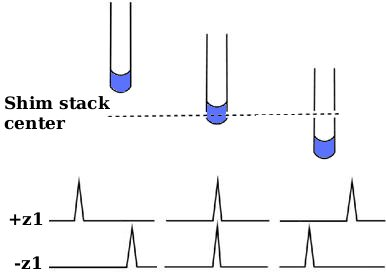When sample is centered in the middle of shim stack, peak position will not depend on value of z1 shim, otherwise peak will move when z1 shim is changed. (Image provided by Jeff Simpson)

z1 shim adds a linear magnetic field gradient gz1 to the main field B0.

Bz1 component arising due to that gradient has the form: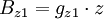$\displaystyle B_{z1} = g_{z1} \cdot z$, where: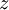$\displaystyle z$ is the coordinate along the axis of magnet relative to the center of shim stack.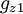$\displaystyle g_{z1}$ is proportional to the value of z1 shim setting

So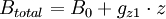$\displaystyle B_{total} = B_0 + g_{z1} \cdot z$

If sample is placed exactly at z=0,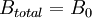$\displaystyle B_{total} = B_0$ and spectrum will not be shifted regardless of$\displaystyle g_{z1}$ (see figure).

However if the sample is at some height z above or below center of shim stack, magnetic field at the center of sample will be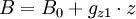$\displaystyle B = B_0 + g_{z1} \cdot z$ and position of peaks will depend on the value of z1 shim.

We can now find the probe position at which sample is located exactly at the center of shim stack by moving the probe up and down until chemical shift of NMR signal is independent of z1 shim setting.

## Sample

Small 100-200 μL sample of H2O. Small sample makes line look narrower in the presence of strong z1 field gradient. Spectra will be recorded unlocked, therefore adding D2O is not necessary.

## Procedure

1. using sample centering tool insert sample into the spinner so that middle of the sample lines up with center of probe when inserted into the magnet.
2. place probe into the magnet at certain depth D.
3. set z1 shim at maximum (positive value) and record 1D spectrum (spectrum 1); find frequency position (F1) at center of mass of the water peak (at 50% peak integral) and take a note of it
4. set z1 shim at minimum (negative value) and record 1D spectrum (spectrum 2); find new peak center of mass (F2) and take a note of it
5. repeat steps 2-4 at series of depths
6. plot (F1 vs D) and (F2 vs D)
7. correct probe depth will be at the intersection of the two curves

Shapes of the two profiles will also give qualitative information on the changes needed to the higher-order z shims.

## Thanks

Thanks for the help putting this together to: Guy Bernard, Gareth Morris, Jeff Simpson, Jeff Walton, Charles Fry, Woody Conover.